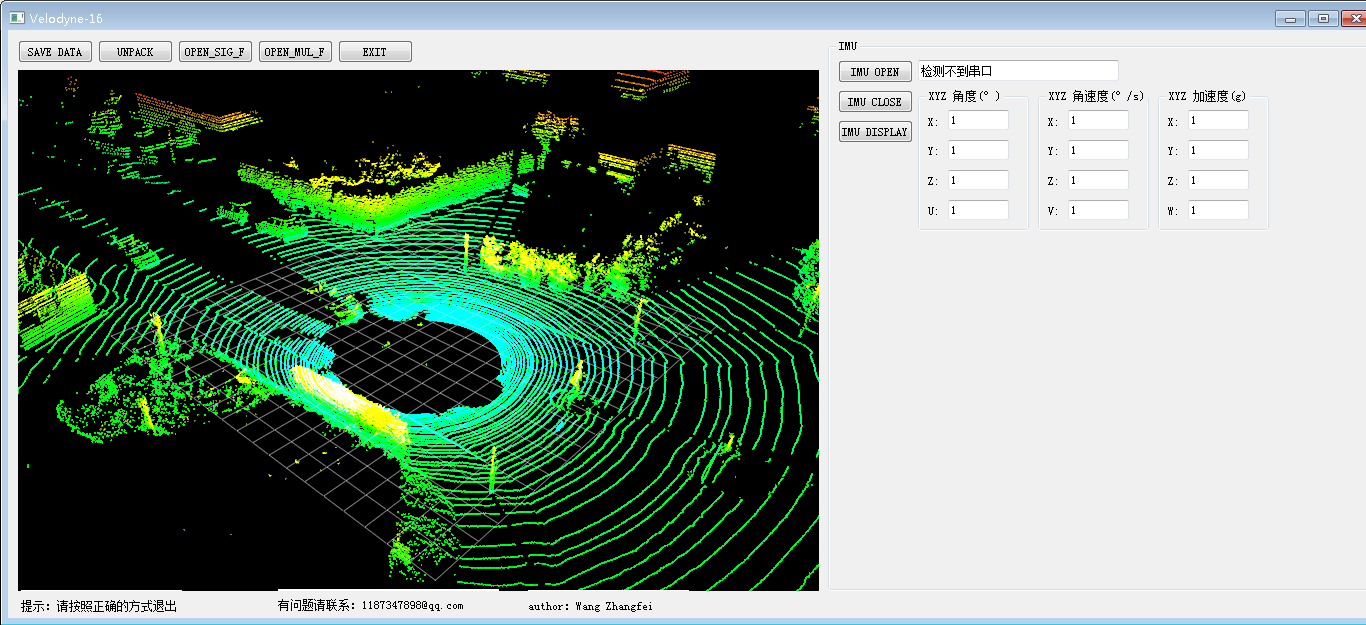## ROS试验车设计②

772
1
2020年9月11日 10时31分1）IMU惯性导航数据解析。
2）制作上位机进行试验车运动调试。
3）使用ROS实现整车调试（获取试验车搭载的传感器数据）。

## 1、IMU惯性导航数据解析。

#!/usr/bin/env python3
# -*- coding: utf-8 -*-

"""
该例程将发布/wt_imu_topic话题，自定义消息类型wt_imu_topic::wt_imu_message

"""

import os
import sys
import numpy as np
if hasattr(sys, 'frozen'):
os.environ['PATH'] = sys._MEIPASS + ";" + os.environ['PATH']
import time
import serial
from inverse_calculation import sign_10_2_16

import rospy
from car_207.msg import wt_imu_message

data_0 = []
data_1 = []

class CAR_CONTROL_TO_ROS():
def __init__(self):
# ROS节点初始化
rospy.init_node('wtzn_imu_node', anonymous=True)
# 打开imu惯导串口
self.wtzn_imu_open()

# 接收IMU惯导发来的数据，使用msg消息方式

# 创建一个Publisher，发布名为/power12v20ah的topic，消息类型为car_207::power12v_20ah，队列长度10
self.wt_imu_info_pub = rospy.Publisher('/wt_imu_topic', wt_imu_message, queue_size=10)

#设置循环的频率
rate = rospy.Rate(50)
self.idx = 0
while not rospy.is_shutdown():
self.idx += 1
# 	# 按照循环频率延时
rate.sleep()

# 接收IMU数据
global roll, pitch, yaw, tt
global data_0,data_1
while 1:
try:
# time.sleep(0.01)

if data_0 == 0x55 :

if data_1 == 0x53:
# print("data_0, data_1", data_0, data_1)
check_sum = data_rcv + data_rcv + data_rcv + data_rcv + data_rcv + data_rcv + data_rcv + data_rcv + data_0 + data_1
check_sum = check_sum & 0xff
# print(data_rcv, check_sum)
try:
if check_sum == data_rcv:
# print("check_sum, data_rcv", check_sum, data_rcv)
roll = ((data_rcv << 8) | data_rcv) / 32768 * 180
pitch = (data_rcv << 8 | data_rcv) / 32768 * 180
yaw = (data_rcv << 8 | data_rcv) / 32768 * 180
tt = (data_rcv << 8 | data_rcv) / 100
#

wt_imu_msgs = wt_imu_message()
wt_imu_msgs.name = "imu"
wt_imu_msgs.imu_roll = roll
wt_imu_msgs.imu_pitch = pitch
wt_imu_msgs.imu_yaw = yaw

# print(wt_imu_msgs.name, wt_imu_msgs.imu_roll, ywt_imu_msgs.imu_pitchaw, tt)
# 发布消息
self.wt_imu_info_pub.publish(wt_imu_msgs)
rospy.loginfo("Publsh power12v20ah message[%s, %.3f, %.3f, %.3f]",
wt_imu_msgs.name, wt_imu_msgs.imu_roll,
wt_imu_msgs.imu_pitch, wt_imu_msgs.imu_yaw)
except:
pass

except:
pass

# 打开串口
def wtzn_imu_open(self):
try:
self.imu_ser_ = serial.Serial('/dev/ttyUSB3', 9600, timeout=0.15)  # com_kou
print("imu_ser_", imu_ser_)
self.recv()  # 将数据进行解析
except:
try:
if self.imu_ser_.isOpen():
print('imu_ser_ 串口已打开')
else:
print('imu_ser_ 串口未打开')
except:
print('imu_ser_ 检测不到串口')

# 关闭串口
def imu_ser_close(self):
try:
self.imu_ser_.close()
except:
print('imu_ser_ 串口已关闭')

# 全部退出程序
def quit(self):
self.imu_ser_.close()
os._exit(0)

if __name__ == '__main__':
try:
car_control_full = CAR_CONTROL_TO_ROS()
except rospy.ROSInterruptException:
pass


msg/wt_imu_message.msg中定义如下：

string name
float32 imu_roll
float32 imu_pitch
float32 imu_yaw


cd..


roscore


source devel/setup.bash
rosrun wt_imu_publisher.py


## 2、制作上位机进行试验车运动调试。

1）四个电机时而不同步，造成试验车不按规定的路线行走（可以微调）。
2）试验车原地转圈会产生共振，造成车辆整体震动，并且震动幅度较大（也有可能是试验车上面我们设计的机构不合理造成的），后面需要进行优化。
3）试验车承重能力较弱（5.5Kg），容易造成超重。## 3）使用ROS实现整车调试（获取试验车搭载的传感器数据）。1）更换试验车底盘，对试验车底盘进行重新优化设计，提高最大承载能力，加装显示器。

2）采用远程控制的方式。

### 评论列表（1条）

•m2apq_6879 2020年10月14日 下午11:05

你好，你四个轮子用的控制器是什么哈，是ros里面的包嘛，如diff_controller